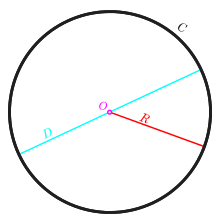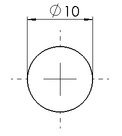# Diameter

For the notion of diameter in graph theory, see Distance (graph theory). For the computer network protocol, see Diameter (protocol).
Not to be confused with Demeter.Circle with circumference (C) in black, diameter (D) in cyan, radius (R) in red, and centre or origin (O) in magenta.

In geometry, a diameter of a circle is any straight line segment that passes through the center of the circle and whose endpoints lie on the circle. It can also be defined as the longest chord of the circle. Both definitions are also valid for the diameter of a sphere. The word "diameter" is derived from Greek διάμετρος (diametros), "diameter of a circle", from δια- (dia-), "across, through" + μέτρον (metron), "measure". It is often abbreviated DIA, dia, d, or .

In more modern usage, the length of a diameter is also called the diameter. In this sense one speaks of the diameter rather than a diameter (which refers to the line itself), because all diameters of a circle or sphere have the same length, this being twice the radius r.For a convex shape in the plane, the diameter is defined to be the largest distance that can be formed between two opposite parallel lines tangent to its boundary, and the width is defined to be the smallest such distance. Both quantities can be calculated efficiently using rotating calipers. For a curve of constant width such as the Reuleaux triangle, the width and diameter are the same because all such pairs of parallel tangent lines have the same distance.

For an ellipse, the standard terminology is different. A diameter of an ellipse is any chord passing through the midpoint of the ellipse. For example, conjugate diameters have the property that a tangent line to the ellipse at the endpoint of one of them is parallel to the other one. The longest diameter is called the major axis.

## Generalizations

The definitions given above are only valid for circles, spheres and convex shapes. However, they are special cases of a more general definition that is valid for any kind of n-dimensional convex or non-convex object, such as a hypercube or a set of scattered points. The diameter of a subset of a metric space is the least upper bound of the set of all distances between pairs of points in the subset. So, if A is the subset, the diameter is

sup { d(x, y) | x, yA } .

If the distance function d is viewed here as having codomain R (the set of all real numbers), this implies that the diameter of the empty set (the case A = ∅) equals −∞ (negative infinity). Some authors prefer to treat the empty set as a special case, assigning it a diameter equal to 0, which corresponds to taking the codomain of d to be the set of nonnegative reals.

For any solid object or set of scattered points in n-dimensional Euclidean space, the diameter of the object or set is the same as the diameter of its convex hull. In medical parlance concerning a lesion or in geology concerning a rock, the diameter of an object is the supremum of the set of all distances between pairs of points in the object.

In differential geometry, the diameter is an important global Riemannian invariant.

In plane geometry, a diameter of a conic section is typically defined as any chord which passes through the conic's centre; such diameters are not necessarily of uniform length, except in the case of the circle, which has eccentricity e = 0.

## Diameter symbolSign ⌀ in a technical drawingNot to be confused with the Scandinavian letter "Ø", the empty set symbol "" or the greek letter phi (Φ).

The symbol or variable for diameter, ⌀, is similar in size and design to ø, the Latin small letter o with stroke. In Unicode it is defined as U+2300 DIAMETER SIGN (HTML &#8960;). On an Apple Macintosh, the diameter symbol can be entered via the character palette (this is opened by pressing ⌥ Opt⌘ CmdT in most applications), where it can be found in the Technical Symbols category.

The character will sometimes not display correctly, however, since many fonts do not include it. In many situations the letter ø is an acceptable substitute, which in Unicode is U+00F8 ø (HTML &#248; · &oslash;). It can be obtained in UNIX-like operating systems using a Compose key by pressing, in sequence, Composedi and on a Macintosh by pressing ⌥ Opt O (the letter o, not the number 0).

In Microsoft Word the diameter symbol can be acquired by typing 2300 and then pressing Alt+X.

In LaTeX the diameter symbol can be obtained with the command \diameter from the wasysym package.

The diameter symbol is distinct from the empty set symbol ∅, from an (italic) uppercase phi Φ, and from the Nordic vowel Ø.